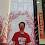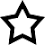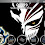## Introduction

Hi! My name is Daniel Lim Jhao Jian. Here is where I share my experience, knowledge and ideas. You are welcome to leave comments and follow my blog. You are free to copy anything from this blog. Please recommend this blog to your friends.

## Monday 30 September 2013

### Tips for SPM Mathematics and Additional Mathematics

For my SPM in year 2012, I got A+ for both Mathematics and Additional Mathematics. Here, I am sharing the tips for SPM Mathematics and Additional Mathematics. These tips are intended for Form 4 and Form 5 students.

Starting from SPM 2021, there are some changes to the examination format for Mathematics and Additional Mathematics, but the tips here are still applicable. Mathematics and Additional Mathematics will continue to be set bilingually in English and Malay for SPM 2021 and beyond, and all students can still choose to answer in English or Malay.

Tips for other SPM subjects can be found here:
http://daniellimjj.blogspot.com/2013/06/complete-tips-for-spm-examination-first.html

SPM MATHEMATICS

1. Studying tips
- When your teacher is teaching in class, pay attention to what your teacher says. Make sure you understand everything taught by your teacher. If you do not understand anything, ask your teacher or friends.
- You need a reference book because the textbooks are not enough. I recommend you to use the Success Mathematics SPM reference book by Oxford Fajar.
- When studying a chapter for the first time, read through the notes in the reference book. Make sure that you understand the concept for that chapter and relate them to what your teacher had taught in school.
- You need to know how to apply what you have learnt to solve questions. Go through all the example questions in the revision book. Make sure that you understand how every question is solved.
- Then, you have to do a lot of exercises. Buy exercise books from the bookshop. You can start by doing topical questions topic by topic. When you have understood all topics, proceed with doing SPM model test papers, past year SPM trial questions and past year actual SPM questions. They should be available from bookshops. After doing the exercises, refer to the suggested answer or mark scheme and do self marking.
- You should sometimes refer to the mark scheme of past year SPM trial papers to find out how every question is marked and which steps need to be shown for every question. Usually, the same types of questions will have similar mark scheme, so this can help you when answering exam questions.
- When doing revision before exam, you should go through the example questions for all chapters in the reference book. If you have forgotten the concept for any chapter, go through the notes in reference book for that chapter again. You should also do some SPM model test papers or past year papers.
- The SPM Mathematics paper will also test on some Form 1 to Form 3 topics in addition to Form 4 and Form 5 topics. Make sure that you do not forget those topics. The Success Mathematics SPM reference books contains some example questions for the Form 1 to Form 3 topics, so you should also go through them.

2. Paper 1
- You do not need to show any workings since the questions are objective questions. Write any rough workings on the question paper if you need to. Solve the question and choose the correct answer among the 4 options.

3. Paper 2
- From SPM 2021 onwards, there are Section A, Section B and Section C. Section A consists of 10 questions where you must answer all questions, Section B consists of 5 questions where you must answer all questions while Section C consists of 2 questions where you can choose any 1.
- You must show all workings for every question. You should not skip any important steps. You do not need to write down the formula used to solve the question. The number of marks allocated for a question usually shows the amount of working needed. 1 mark will be given for each important step and the final answer. Marks can only be given for the final answer if the marks for working are scored. If you do not show a particular important step, marks may not be given for that step, as well as all important steps after that step and the final answer, even if they are correct. Therefore, correct answer without working usually scores 0 mark. On the other hand, if your final answer is wrong but some of your working is correct, you may still be given some marks.
- You are not allowed to write extra solutions or answers for any question. If you do so and any of the answers/solutions is wrong, marks will be deducted. When copying figures from the question or from your answer for the previous part of the question, be careful not to copy wrongly.
- If the question does not state that you must or you cannot use a particular method, then you can use any suitable method to solve the question. You can also use methods that are learnt only in Additional Mathematics or in STPM / A Level or higher level, if you know them. If the question states that you must use a particular method, then you can only that method to solve the question. If the question states that cannot use a particular method, then you cannot that method to solve the question
- If the final answer is a number with infinite decimal places, you should round up to at least 4 significant figures.You can also write your final answer as a fraction if possible. However, if the question states that you must express your answer as fraction or decimal, then you must follow the instruction. If a number with infinite decimal places is involved in between the workings, you should take at least 5 significant figures (4 significant figures is not sufficient) to ensure accuracy of the final answer. Otherwise, marks can be deducted if the final answer is less accurate.
- For questions involving Pi, the question may ask you to use Pi = 22/7. In that case, you cannot use Pi = 3.142 or the value of Pi from the calculator. You must also follow other instructions given in the question.

4. Both Paper 1 & Paper 2
- When answering, read the question and information given carefully. For questions involving diagrams, mark any important details on the diagram. For some questions without diagram, sometimes it may be helpful to draw a diagram so that you can illustrate the information given in the question. Apply what you have learnt in mathematics in order to solve the questions and get the answer. Use the correct concept or formula for every question.
- Certain formula are provided in the first few pages of the question paper, refer to them if necessary. After you have answered all questions, you should recheck your answers for mistakes. Leave at least 15 minutes to recheck your answers if possible. When rechecking, use a different method to solve the question or directly use the calculator if possible.

1. Studying tips
- When your teacher is teaching in class, pay attention to what your teacher says. Make sure you understand everything taught by your teacher. If you do not understand anything, ask your teacher or friends.
- You need a reference book because the textbooks are not enough. I recommend you to use the MasterClass SPM Additional Mathematics reference book by Sasbadi.
- When studying a chapter for the first time, read through the notes in the reference book. Make sure that you understand the concept for that chapter and relate them to what your teacher had taught in school.
- You need to know how to apply what you have learnt to solve questions. Go through all the example questions in the revision book. Make sure that you understand how every question is solved.
- Then, you have to do a lot of exercises. Buy exercise books from the bookshop. You can start by doing topical questions topic by topic. When you have understood all topics, proceed with doing SPM model test papers, past year SPM trial questions and past year actual SPM questions. They should be available from bookshops. After doing the exercises, refer to the suggested answer or mark scheme and do self marking.
- You should sometimes refer to the mark scheme of past year SPM trial papers to find out how every question is marked and which steps need to be shown for every question. Usually, the same types of questions will have similar mark scheme, so this can help you when answering exam questions.
- When doing revision before exam, you should go through the example questions for all chapters in the reference book. If you have forgotten the concept for any chapter, go through the notes in reference book for that chapter again. You should also do some SPM model test papers or past year papers.
- The Additional Mathematics topics may require knowledge and understanding of the Mathematics topics. Make sure that you understand all the topics in SPM Mathematics.

2. Both Paper 1 & Paper 2
- From SPM 2021 onwards, Paper 1 has Section A and Section B while Paper 2 has Section A, Section B and Section C. For Paper 1, Section A consists of 12 questions where you must answer all questions while Section B consists of 3 questions where you can choose any 2. For Paper 2, Section A consists of 7 questions where you must answer all questions, Section B consists of 4 questions where you can choose any 3 while Section C consists of 4 questions where you can choose any 2.
- When answering, read the question and information given carefully. For questions involving diagrams, mark any important details on the diagram. For some questions without diagram, sometimes it may be helpful to draw a diagram so that you can illustrate the information given in the question. Apply what you have learnt in mathematics in order to solve the questions and get the answer. Use the correct concept or formula for every question. Certain formula are provided in the first few pages of the question paper, refer to them if necessary.
- You must show all workings for every question. You should not skip any important steps. You do not need to write down the formula used to solve the question. The number of marks allocated for a question usually shows the amount of working needed. 1 mark will be given for each important step and the final answer. Marks can only be given for the final answer if the marks for working are scored. If you do not show a particular important step, marks may not be given for that step, as well as all important steps after that step and the final answer, even if they are correct. Therefore, correct answer without working usually scores 0 mark. On the other hand, if your final answer is wrong but some of your working is correct, you may still be given some marks.
- You are not allowed to write extra solutions or answers for any question. If you do so and any of the answers/solutions is wrong, marks will be deducted. When copying figures from the question or from your answer for the previous part of the question, be careful not to copy wrongly.
- For a part of question which requires you to use your answer from the previous part, even if your answer for the previous part is wrong and you use it for this part, causing your answer for this part to be wrong, you will still get the working marks for this part as long as your calculation for this part is correct, but you will usually lose the answer marks for this part.
- If the question does not state that you must or you cannot use a particular method, then you can use any suitable method to solve the question. You can also use methods that are learnt only in STPM / A Level or higher level, if you know them. If the question states that you must use a particular method, then you can only that method to solve the question. If the question states that cannot use a particular method, then you cannot that method to solve the question.
- If the final answer is a number with infinite decimal places, you should round up to at least 4 significant figures. You can also write your final answer as a fraction if possible. However, if the question states that you must express your answer as fraction or decimal, then you must follow the instruction. If a number with infinite decimal places is involved in between the workings, you should take at least 5 significant figures (4 significant figures is not sufficient) to ensure accuracy of the final answer. Otherwise, marks can be deducted if the final answer is less accurate.
- For questions involving Pi, the question may ask you to use Pi = 3.142. In that case, you cannot use Pi = 22/7 or the value of Pi from the calculator. You must also follow other instructions given in the question.
- After you have answered all questions, you should recheck your answers for mistakes. Leave at least 15 minutes to recheck your answers if possible. When rechecking, use a different method to solve the question or directly use the calculator if possible.

Tips for other SPM subjects can be found here:
http://daniellimjj.blogspot.com/2013/06/complete-tips-for-spm-examination-first.html

1.Hai Daniel Lim, Can you help me how to know which formula to answer for add math. For example, the quadratic equation form 4 with a lot of formula.....how do you know to use formula which one.

1.Hi, with regards to your question, from what I remembered, there is one formula used to calculate the roots of the curve but I don't remember there are many formulas for this chapter. Anyway, if you need help on Math and Add Math, you can PM me through FB (music.gerald.tan@gmail.com) and give me a clearer picture of your question. I would be happy to help you on that :)

2.When finish form five , we had to make a choice whether matripulasi or college or university .... which is better ?

1.http://daniellimjj.blogspot.com/2013/01/after-spm-plans-to-be-continued.html

3.Hey, I'm taking spm this year and was just wondering which questions i should choose to do for paper 2 of both add math and math papers! The selective questions, eg. Index, solution of triangles, longitude latitude etc. Thanks :)

1.Hi there. I'm also an spm 2014 student. I would Suggest index and solution of triangles because the formulas and concepts are easy to understand :) . Motion along the straight line is also okay, but be wary of how the questions twist. :) as for maths, try answering everything.

2.For Maths, I would suggest that you do all the 5 selective questions if you have time. For Add Maths, I would advise you to choose Index Number and Motion Along a Straight Line. You can also choose Linear Programming if you understand it. Avoid Solutions of Triangles if possible because the calculations are usually quite long and it is easy to make mistakes.

4.hello, im spm candidate this year . so i want to ask how to study addmath for last minute ? the express one :)

1.Practise past year. It should work wonders

5.Why did you mostly use oxford fajar as a reference since there are many more publisher. Pls give me a good reason since you are an excellent student

1.Because they contain everything in the SPM syllabus and the explanations are clear and detailed.

6.Hi Daniel, I would like to ask whether you know the weightage of marks allocated for the Modern Maths paper....for eg is it like 35/40 (Paper 1) + 80/100 (Paper 2) = 115/140 marks and then this mark is brought to 115/140X100 = 82%? Or is there a higher weightage given for Paper 2? Thanks

1.The weightage is like what you said, 40/140 for Paper 1 and 100/140 for Paper 2. There is no higher weightage for Paper 2.

2.Thanks very much Daniel and all the best to you =)

7.Is it OK to use nexus bijak Mathematics reference book?

1.Hi ! :D

Yeah, there's no problem to use it, as not all the reference books are suited for all students. From my side of view, the ellaborations on the points in reference books are almost the same, especially for Maths and Add Maths. Meanwhile, they might be vary in examples given and exercises. Just feel free to choose the one that you comfort with.
:)

8.This comment has been removed by the author.

9.Hi, I love to study addmath and physics and I'm starting to study a levels syllabus just for extra knowledge. However I feel lazy to study modern maths, how to overcome this?

10.This comment has been removed by the author.

11.Hi Daniel :) i have a question. i get 79/80 for paper 1 addmath. How many marks i must get for paper 2 in order to get A+ for addmath? btw, thank you for sharing these greatful tips!

1.kira la sendiri.

12.Thanks for sharing an informative article. I am searching aboutLinear Motion Share some about it.